@吃饭第一名的 Claire

“数据人创作者联盟”成员。

00

• 请读者原谅笔者中英混杂的文风和日渐退化的中文表达

• 本文中的一些测试题最好先思考、再看答案哦

01

• 如果所有的数都只出现了一次，那就没有众数，如果两个值同时出现最高频次，就有2个众数，这就是著名的bimodal distribution（比如消费者在餐厅的就餐时间就遵循这样的分布，如下图）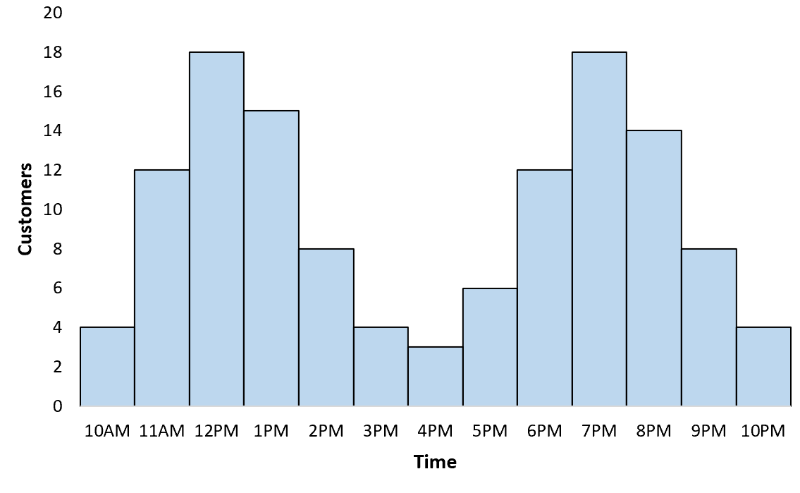Note: A bimodal distribution is a probability distribution with two modes（众数）.

• 中位数是排序后找位置(n+1)/2的数，如果结果是x.5就要找x和x+1位置的数，取平均值

• 中位数是80意味着有一半的数比80大

• 平均数有它的局限性，以下3组数的平均数相同，但明显是很不一样特征的数，所以我们还要看一个dataset的标准差(standard deviation)以表示数据的variability（变化）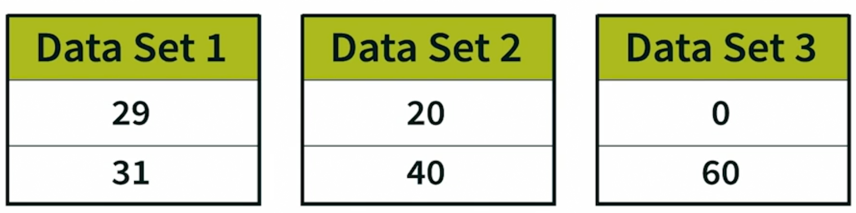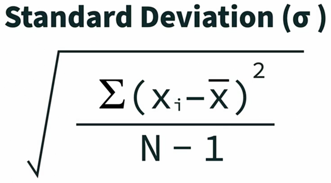• 补充一下离散型随机变量的标准差算法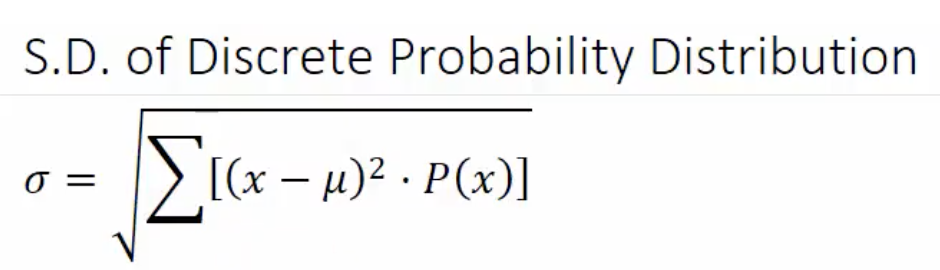其中mu是加权平均值，P(x)是x出现的频率。举例：消费者在商店A一周的消费次数如下表，最后该店消费者平均一周消费1.72次，标准差是1.01次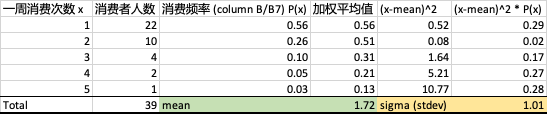• Z-score:用标准差来衡量一个数离平均值的距离（适用于正态分布的情况，后面会和t-statistics以及t-distribution进行区分）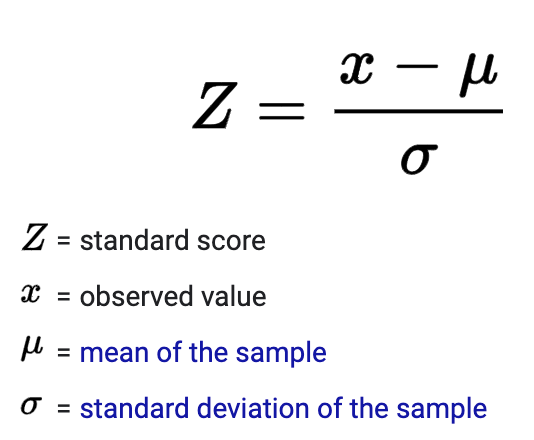例子：美国数据分析师的平均年薪\$100,000, 标准差\$20,000, Lucy年薪\$60,000,她的年薪的z-score是多少呢？

(60-100)/20= - 2，也即比全国平均水平低2个标准差

• 经验法则/ 3-sigma法则empirical rule = 68–95–99.7 rule适用于对称的正态分布的数据，它的意思是68%的数据在平均值+-1个标准差的范围内，95%的数据在平均值+-2个标准差的范围内（准确来说是1.96个），99.7%的数据在平均值+-3个标准差的范围内。这3个数据能让你在算出你的z-score后更快的理解你的数据所处的位置。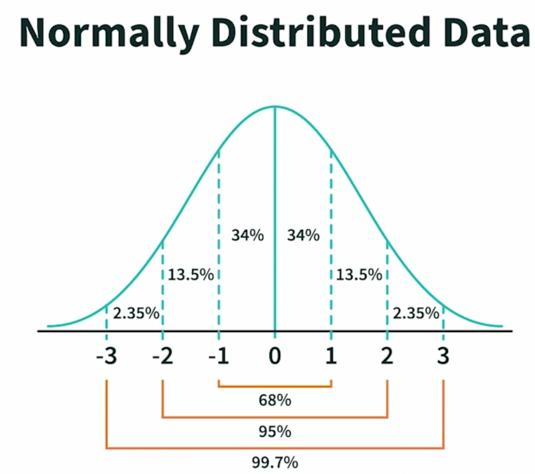02

• 有8个选手参赛，只给前3颁奖，前三名的可能性有多少？

注意这里顺序重要，所以用排列。A38 = 8X7X6= 336种

• 12个人里面随机选4个人参赛，Lily和Lucy想一起参赛，Lily和Lucy一起参赛的probability是多少？

分母：所有可能的结果 = C412 = (12X11X10X9)/(4X3X2X1) = 495

分子：期望的结果 = C210 = 10X9/(2X1) = 45 （已经确定Lily和Lucy会参加，所以是从剩下的10个人里选2个）

• Nth percentile的意思是n%的数低于这个值。

The 25th percentile is also called the first quartile（一分位数）.

The 50th percentile is the median

The 75th percentile is also called the third quartile.

The difference between the third and first quartiles is the interquartile range.

Percentile的计算公式 (#values below + 0.5)/ total #of values X 100

(60-45-1)+0.5/60 = 24% -> 24th percentile

• 多事件概率

a. 遇到either or（多种情况）的事件，可以考虑1-补集的概率。

P(你赢的概率) = 1-P(你输的概率) = 1-P(两次都是背面的概率) = 1- ½ * ½ = ¾

Note:这里用到了独立事件的可乘性

b. 贝叶斯定理：描述在已知一些条件下，某事件的发生机率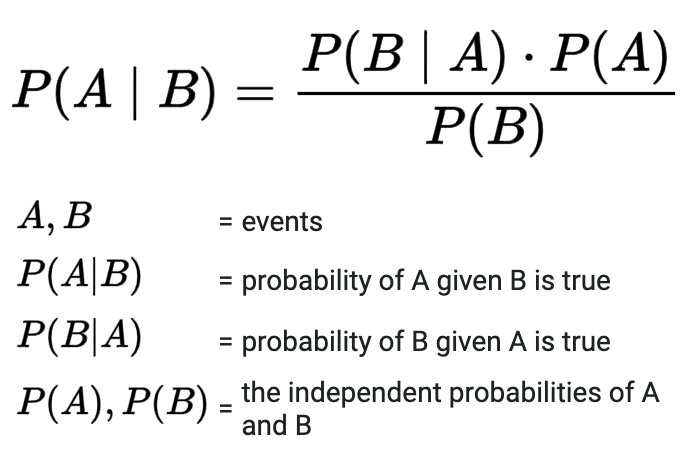Event A: 实际没病 no disease

Event B: 被测出有病test positive

P(B|A) = 2%

P(A) = 1-1/10000= 99.99%

P(B) = 0.01% * 99% + 99.99% * 2% = 2.0097%

P(A|B) = 99.99% * 2%/2.0097% = 99.5%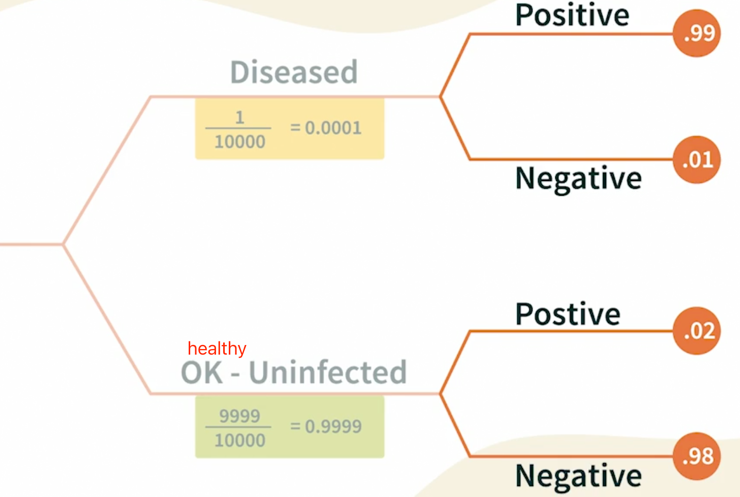c. Binomial distribution二项分布是离散概率分布的一种，求n个独立事件中x次成功的概率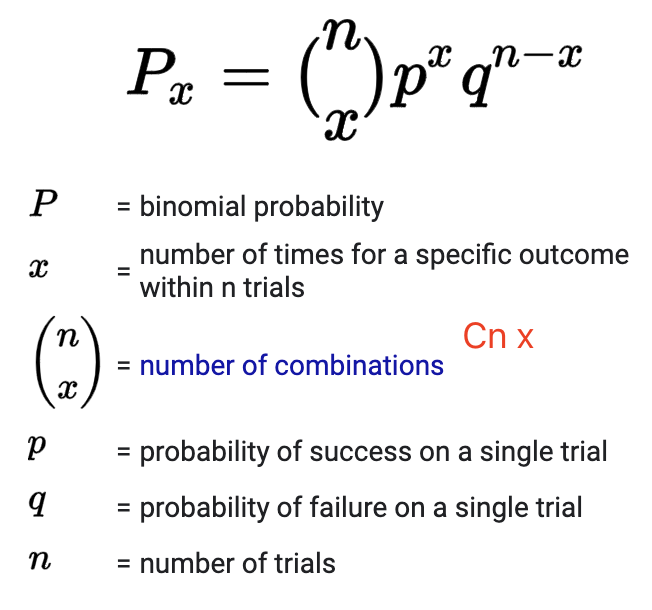4个人的组，朋友的组合是C42 = 6种，没趣的情况就是6种组合都是朋友，如果N是一个组中朋友的对数，N就符合二项分布 N~bin(6,1/2) (6个独立组合里，是朋友的概率是1/2)，没趣的概率就是(1/2)^6，有趣的概率就是1-1/64= 63/64.

03

• 先明确sample proportion （样本比例）and sample mean（样本均值）的区别

Sample proportion: 一群数据中某特征的比例

p̂ = x / n

•x: 样本中具备某特征的数据

•n: 样本中所有数据点

Sample mean: 样本的平均值

x = Σxi / n

• 中心极限定理：随着样本个数和每个样本中的数据量增加，sample mean会接近population mean，sample proportion会接近population proportion

• 已知总体比例(population proportion)求样本比例区间。问：国家手机统计报告表示60%的人对自己的手机提供商表示满意，如果我取100个人为样本， 68%的随机样本里会有%多少到%多少的人对自己的手机提供商表示满意？

• 根据之前提到的empirical rule，68%的随机样本包含了离population proportion 1个标准差的的样本 - 1 standard deviation of the population proportion，题目中60%是population proportion，standard deviation of the population proportion的公式是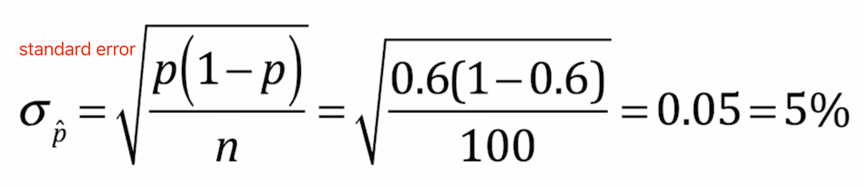Note: standard deviation of the population proportion也叫standard error

• 置信区间Confidence interval。是通过一个样本来推测总体均值/比例在95% confident的情况下落在哪个区间。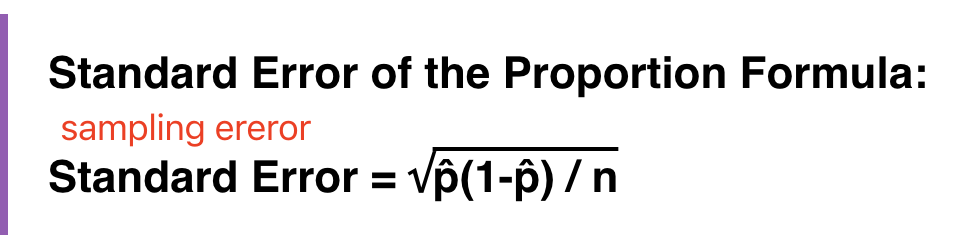• 假设检验hypothesis test

第一步建立假设，注意H0(null hypothesis)是现状，是没差别，Ha (alternative hypothesis)是事情有改变，或者你想测试的东西。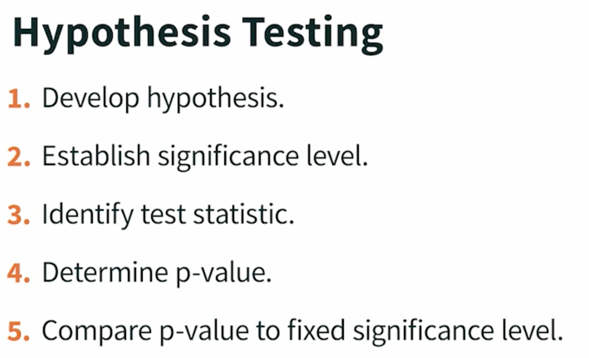假设检验的关键之一是选择统计指标，

H0: p<= 0.5 （Lily落选）

Ha: p> 0.5 （Lily当选）

z = (x – μ) / σ = (0.54-0.5)/ sqrt(0.5*(1-0.5)/500) = 1.79

• 其中 μ (the population mean) and σ (the population standard deviation)

• population proportion的standard deviation是sqrt(0.5*(1-0.5)/500)